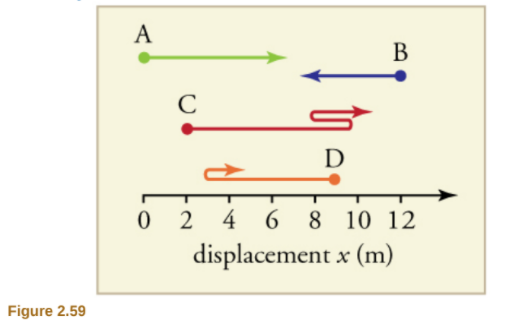×
Get Full Access to College Physics - 1 Edition - Chapter 2 - Problem 1pe
Get Full Access to College Physics - 1 Edition - Chapter 2 - Problem 1pe

×

# Find the following for path A in Figure 2.59: (a) TheISBN: 9781938168000 42

## Solution for problem 1PE Chapter 2

College Physics | 1st Edition

• Textbook Solutions
• 2901 Step-by-step solutions solved by professors and subject experts
• Get 24/7 help from StudySoup virtual teaching assistantsCollege Physics | 1st Edition

4 5 1 297 Reviews
15
1
Problem 1PE

Find the following for path A in Figure 2.59: (a) The distance traveled. (b) The magnitude of the displacement from start to finish.  (c) The displacement from start to finish.Step-by-Step Solution:

Solution 1PE

Step 1 of 4

For the given path A in below figure, we need to calculate the distance travelled, magnitude of the displacement from start to finish and the displacement from start to finish.In the given case, the object is moving along x-direction initially from positionto final positionand covers a total distance along the path is.

Step 2 of 4

Step 3 of 4

##### ISBN: 9781938168000

The full step-by-step solution to problem: 1PE from chapter: 2 was answered by , our top Physics solution expert on 07/07/17, 04:39PM. College Physics was written by and is associated to the ISBN: 9781938168000. This full solution covers the following key subjects: displacement, start, finish, Find, figure. This expansive textbook survival guide covers 34 chapters, and 3125 solutions. The answer to “?Find the following for path A in Figure 2.59: (a) The distance traveled. (b) The magnitude of the displacement from start to finish. (c) The displacement from start to finish.” is broken down into a number of easy to follow steps, and 30 words. Since the solution to 1PE from 2 chapter was answered, more than 387 students have viewed the full step-by-step answer. This textbook survival guide was created for the textbook: College Physics , edition: 1.

Unlock Textbook Solution